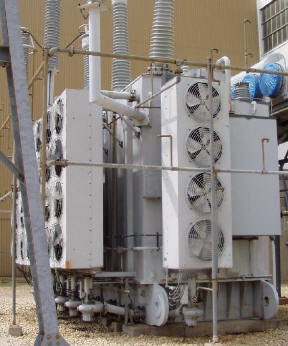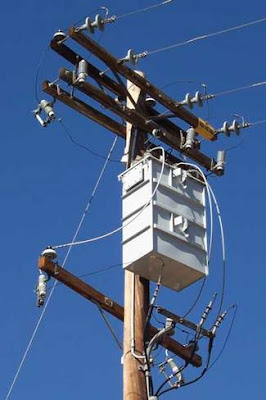## Tuesday, February 9, 2010

### Step-up Transformer and Step-down Transformer

Step-up TransformerIn a step-up transformer the potential difference across the secondary coil is greater than the potential difference across the primary coil.
Step-Up Transformers are one of the really common and vital electrical tools used in power transmission and modification. They are usually the first major transformer in a transmission system and are often used in various forms throughout the system.

Step-Up Transformers are based on the same formulas that other transformers are, but the key element is that they convert voltages to higher volumes while reducing amperage and effects of resistance. This makes Step-Up Transformers ideal in long-distance power transmission use; by stepping up voltage and reducing amperage little energy is lost to resistance.

Step-down TransformerStep down transformers are designed to reduce electrical voltage. Their primary voltage is greater than their secondary voltage. This kind of transformer "steps down" the voltage applied to it. For instance, a step down transformer is needed to use a 110v product in a country with a 220v supply.

Step down transformers convert electrical voltage from one level or phase configuration usually down to a lower level. They can include features for electrical isolation, power distribution, and control and instrumentation applications. Step down transformers typically rely on the principle of magnetic induction between coils to convert voltage and/or current levels.

Step down transformers are made from two or more coils of insulated wire wound around a core made of iron. When voltage is applied to one coil (frequently called the primary or input) it magnetizes the iron core, which induces a voltage in the other coil, (frequently called the secondary or output). The turns ratio of the two sets of windings determines the amount of voltage transformation.

An example of this would be: 100 turns on the primary and 50 turns on the secondary, a ratio of 2 to 1.

Step down transformers can be considered nothing more than a voltage ratio device.

With step down transformers the voltage ratio between primary and secondary will mirror the "turns ratio" (except for single phase smaller than 1 kva which have compensated secondaries). A practical application of this 2 to 1 turns ratio would be a 480 to 240 voltage step down. Note that if the input were 440 volts then the output would be 220 volts. The ratio between input and output voltage will stay constant. Transformers should not be operated at voltages higher than the nameplate rating, but may be operated at lower voltages than rated. Because of this it is possible to do some non-standard applications using standard transformers.

Single phase step down transformers 1 kva and larger may also be reverse connected to step-down or step-up voltages. (Note: single phase step up or step down transformers sized less than 1 KVA should not be reverse connected because the secondary windings have additional turns to overcome a voltage drop when the load is applied. If reverse connected, the output voltage will be less than desired.)

So in a step-up transformer the potential difference across the secondary coil is greater than the potential difference across the primary coil while in a step-down transformer the potential difference across the secondary coil is less than the potential difference across the primary coil.

Image and Information Source: www.goggle.com